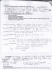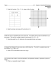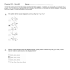# Electric Field

## Transcription

Electric Field
```Physics 122 – Class #13 – Outline
Announcements
●Coulomb's Law
●Electric Field
● Superpositions
●
Next time ● Empirical Electrostatics
● Field of continuous distributions
●
Reading – Last Week
ALL of Chapter 25 …
It is key to rest of course.
This Week – Chapter 26
You may skip 26.7
Actively read the examples in the text … particularly in 26.2 and 26.3
Main Concepts – Ch 25.
Coulomb's Law, Charge, Electric Field
Applies to lab
Reading Assignment (next class)
Read Chapter 26 (Electric Field)
Read through section 26.3.
In 26.4, skip disk of charge but read plane of charge and sphere of charge. 26.5 and 26.6 are important. Skip 26.7.
(We will skip Chapter 27 and continue w/ Chapter 28)
How to read Knight
He usually starts each chapter with a section on “empirical observations”, giving you an intuitive idea of a new electrical concept. He then proceeds to the math and the models.
While you are predominantly tested on math and models … you need the empirical observations to make sense of it all (Particularly if you have never had a physics course in electricity or magnetism).
Coulomb's Law – With vectors

F12=k
q1 q2
r
2
12
1
k=
4  0
r
12
9
2
k=9.0×10 N m /C
2
−19
Electron (e)=1.6×10
F 12
Means force that particle one exerts on particle 2.
r 12
Is a unit vector that points from particle 1 to particle 2
C
Steps to solve a superposition problem
1) Identify the charge (or point P) at which you want to calculate the force (or field).
2) Draw an arrow (a vector) representing the Force Vector (or Field Vector) at the charge along a line joining it with each of the other charges.
3) Make the length of the vectors proportional to the force between the charges (shorter arrows for more distant charges)
4) Add the vectors using the tip to tail method to find the resultant.
Superposition problem #1
Estimate the direction and magnitude of the NET force on the central charge due to the other four charges.
Superposition problem #1
Clicker
The net force on –q is
A. Up.
B. Down.
C. Left.
D. Right.
E. The force on –q is zero.
Slide 25-85
Superposition problem #2
Estimate the direction and magnitude of the force on the central charge due to the other four charges.
Superposition problem #2 (similar to written HWs)
Clicker
Which is the direction
of the net force (and field)
on the charge at the top?

E. None of these.
Slide 25-79
The Field Model
 The photos show the
patterns that iron filings
make when sprinkled
around a magnet.
 These patterns suggest
that space itself around
the magnet is filled with
magnetic influence.
 This is called the
magnetic field.
 The concept of such a “field” was first introduced by
Michael Faraday in 1821.
The Field Model
 A field is a function that
assigns a vector to every
point in space.
 The alteration of space
around a mass is called
the gravitational field.
 Similarly, the space
around a charge is altered
to create the electric
field.
Slide 25-88
The Electric Field
If a probe charge (or test
charge) “q” experiences an
electric force at a point in
space, we say that there is
an electric field
at that point causing the
force.

The units of the electric field are N/C. The magnitude E of the
electric field is called the electric field strength.
Slide 25-89
Electric Field
The force/Coulomb felt by an infinitesimal charge from all other charges in the area (not including itself).

F 12 =k
q1 q2
r
2
12
r
12

E 12 =k
q2
r
2
12
r
12

F 12 =q 1 
E 12
Positive charges “emit” electric field lines.
Field lines end on negative charges.
The Electric Field of a Point Charge
 Using unit vector notation,
the electric field at a
distance r from a point
charge q is:
 A negative sign in front of
a vector simply reverses
its direction.
 The figure shows the electric field of
a negative point charge.
Slide 25-100
Clicker
The Electric field at the
location
of –q is
A. Up.
B. Down.
C. Left.
D. Right.
E. The force on –q is zero.
Slide 25-85
Electric Field Magnitudes
Given q=10 nC , r=3 m
N⋅m
k=9×10
2
C
E=?
9
⃗ =k
E
2
2
[ A ] 1N/C
[C] 10 N/C
[B] 3 V / m
[D] 30 N/C
q1
r
2
12
r̂
12
[E] 90 N/C
Using an electric field simulator
/simulation/charges-and-fie
lds
Electric Fields – Why bother? F=q
⃗
⃗
E
Electric fields are measurable.
If you know the field where you are, you know its effects on charges

Complex charge distributions can produce simple electric fields (e.g. line charge).
Time varying electric fields carry energy … we call it radio, or light, or X­rays.


When you look at a star, you see the electric field from a charge that may have produced it a million years ago.
Problem 25.37
```

### MAGNETISM, n. Something acting upon a magnet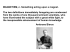### Assignment (02) Introduction to Electric Charges and Electric Field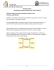### Market Summary Report (BETOS groupings)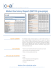### Phys 208 - Recitation E-Fields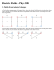### Comp are prop erties o f two fun ctions each repre sented in a differe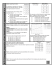### vector - SurfaceWorks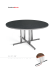### Document 6560231